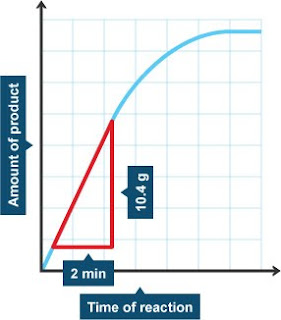## Monday, May 28, 2012

### Tips to Calculate RateOne of the most common and well-known algebraic equations is calculating distance, time or rate, and if you are one of the many persons worldwide that dread algebra, it can be one of the scariest things to encounter on an examination paper or in your daily activities. Calculating distance, time and rate is one of the simplest algebraic problems however, once you know the formula for working it out. First you should know that all three are related and you cannot find one without information about the other two, so you cannot separate them. With that said, let's look at how you go about solving each problem.

• Calculator

• Pen
• Paper

## Instructions

1. The first thing to learn and remember is the basic formula which is that:
2. Distance = Rate x Time
3. Once you know the basic formula then just grab your calculator, punch in the figures and write everything down so you don't forget the answers. If you need to find the distance between two points, you need to know how fast the vehicle will travel between each point (rate) and how long it will take to get from point A to point B traveling at that rate (time). So for example:
4. A car travels at 80mph and drives for 3 hours. Calculate the distance the car traveled.
5. Remember the formula:
Distance = Rate x Time
So Distance = 80mph (rate) x 3 hours (time)
Distance = 240 miles.
6. To calculate rate or time the formula has to be rearranged a bit; to determine how long it will take to get from point A to point B (time) the formula is:
7. Time = Distance/Rate
8. So if you are given the distance (let's say 220 miles) and you know the speed the vehicle will be traveling at (50mph) then you can find how long it will take to travel the given distance at a given rate.
9. Time = Distance/Rate
So Time = 220miles/50mph
Time = 4.4 hours
10. The last one is Rate which follows the same pattern as Time, meaning that you divide to calculate both Time and Rate. So if you know the distance between Point A and B (let's use 220 miles again) and you know the vehicle will get to its destination in 4.4 hours, then it is easy to calculate the rate the vehicle will be traveling at to travel that distance in that number of hours:
11. Rate = Distance/Time
Rate = 220 miles/4.4 hours
Rate = 50mph

## Tips and Warnings

• To make things easier, remember that when calculating Distance you multiply the other two, to calculate Rate or Time the formula is reversed and you divide instead of multiply.
• Always start the formula with Distance to prevent confusion. For instance:
Distance = Rate x Time
Rate = Distance/Time
Time = Distance/Rate
• If you are taking an exam, write down the formula for calculating each thing before you begin the exam so you have something to refer to if you forget the formula.